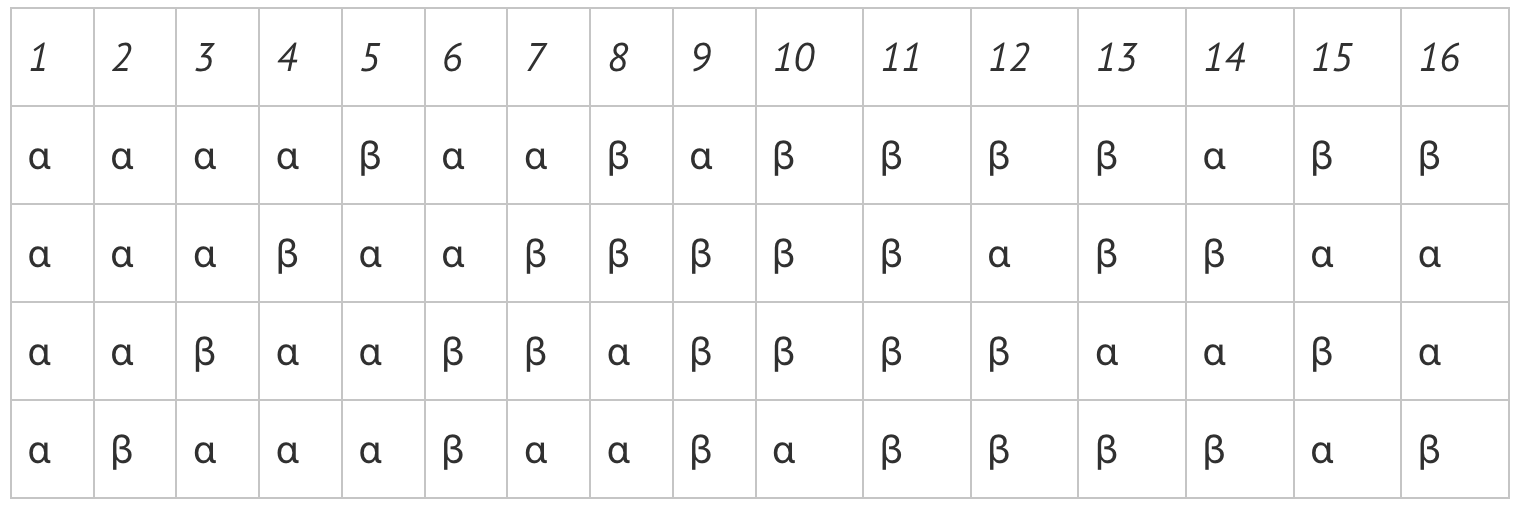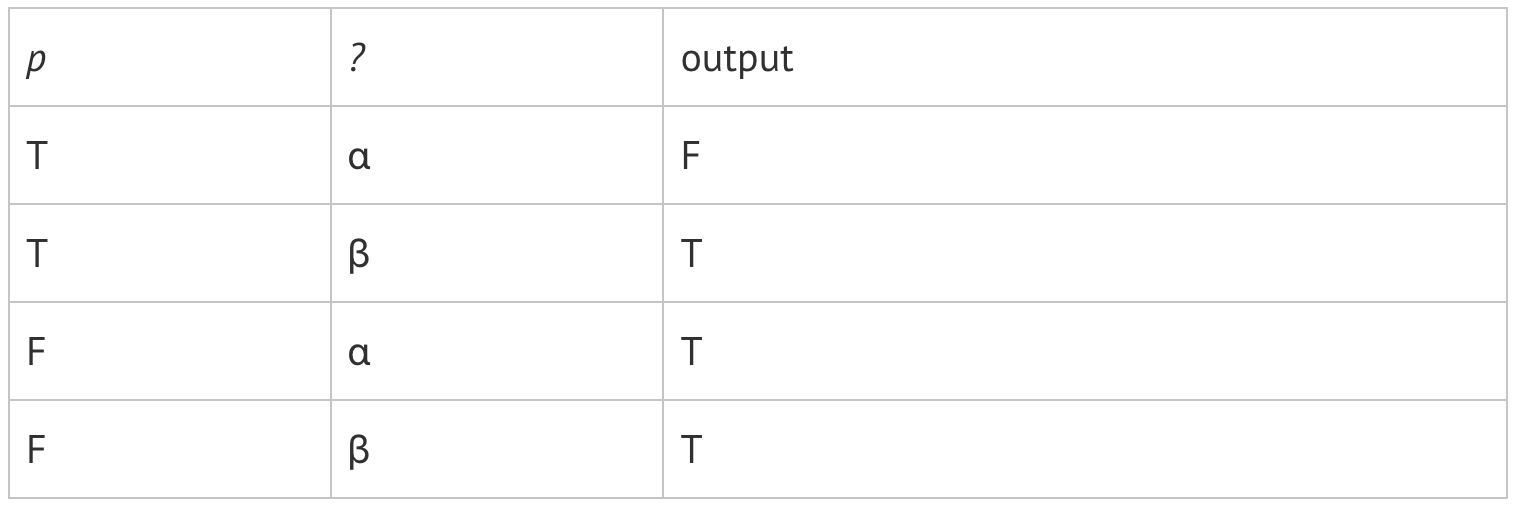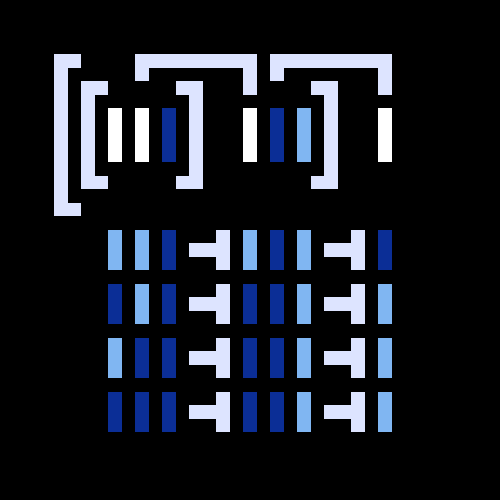From NAND to SKI

Posted on

Last time we looked at the complete list of binary operators on true and false. We constructed one operator that can generate all the others. I’ve purposely obfuscated the graph below to erase any identity of true or false and reordered them in a way to illustrate how the table is every combination of two items in the four slots.I asked previously “have we really done anything that has meaning here?” Without a T or F, the above table is just symbols, void of meaning. We may want to instinctively attach T and F to one of the symbols above but we shouldn’t. By doing so we lose a process that in this post we will try to uncover.

To see the problem, how do I know which symbol should get a T or F? Does it matter? No, as long as I stay consistent in replacing one symbol for T and the other for F, I am guaranteed to have all the operators I would need because the table is exhaustive. I can’t create any new combination.

Now let’s look at an example where that isn’t exactly the case.Suppose someone said that you must change the two greek symbols into T or F to get the correct output, can you do this? We can try putting in T for the first symbol and F for the second. This would suggest our output is NAND and perhaps we are happy with that and decide to move on and read something more interesting.

Or we try F for the first and T for the second and end up with . There is no way to decide which option to take because the system isn’t well defined. The only way to force the choice requires additional information. If the output is told to be NAND or → then we would immediately know what to do. But suppose we aren’t allowed to either name the output or tell you right out what one symbol means, what is the least amount of information I can give you that forces you into picking T for one of the symbols? I’m defining information broadly here and not in the Shannon entropy sense.

If I add T && T => F to the system, I can force you into picking T for the first symbol and the rest falls out, but this is the same as telling you the first symbol is T. So that’s not going to work.

Now what if I said “two of the same type return the other type”. This works given the table above because it will force a choice in the top and bottom line. Then these forced results can be substituted in for the two inner lines. So why is this statement different from T && T => F. For one, true and false are nowhere to be seen! This statement works anonymously. We are allowed to define the symbols it acts on separately. This is a very powerful idea!

We are now going to start making some jumps into computation theory. To those unfamiliar, this may seem daunting, tedious or uninteresting but I promise that it will be rewarding if you stick with me. Also you can click away at anytime.

Computation Theory

We know that NOR and NAND are functionally complete and these abstract gates can be put together in specific orders to run computers. Therefore NOR and NAND represent elements in a system of computation. The theory of computation is to describe precisely what properties allow NOR, NAND and a host of other objects to be computational. The natural question to ask then is what exactly is computation. The Church-Turing Thesis says that any function to be regarded as computable is computable by a Turing machine. The first time I heard this I thought that this is rather a weak assertion and in some sense felt circular. A Turing machine is an abstract model of a computer. It has a long tape and on that tape are symbols in which the head of the machine can read and change based on a finite table. For its simplicity, a Turing machine is incredibly powerful. The Church-Turing Thesis says that if a Turing machine can simulate a function then that function is computable.

So who’s Church? Well Alonzo Church was Alan Turing’s doctoral advisor and created a system known as lambda calculus, λ-calculus, in an exploration of the foundations of mathematics. The system relies on function abstraction, substitution and binding. At first, this system is hard to build intuition on in comparison to Turing’s model. But not only are they equivalent, Church’s model, in my opinion, allows for a deeper understanding of computation.

We are going to look at another calculus called SKI-calculus created by Moses Schönfinkel and Haskell Curry. This system is very much like λ-calculus but I think easier to begin with. For the life of me, I could not find exactly what SKI stands for but we can think of them as standing for substitution, k(c)onstant, and identity. They were probably in german. Each letter represents a combinator, which is a type of function without free variables. It’s a fancy way of saying a function that has to do stuff with what it’s given.

I – identity – takes an expression and returns it.

Ix = x

K – Konstant – takes an expression and returns the first expression back.

Kxy = x

S – Substitution – takes three expressions and applies the second to the third. Takes that result and applies it to the first to the third.

Sxyz: (yz) -> xz(yz)

So S is definitely the weirdest of the bunch so lets try working on one.

SKKx

So we apply our rules and we get:

SKKx -> (Kx) -> Kx(Kx)

Now we know that K will return the first expression. So we can rewrite the internal (Kx) as y to get something in the form as above:

Kx(Kx) -> Kxy

Then reduce to:

Kxy -> x

This is the same as I! Which means that SKK is the same as I. So I is really unnecessary but we like having it around. So why do I bring up this system? Even though there are only three combinators, this system is as powerful as a full fledged Turing machine or full λ-calculus! So how can we make a NAND gate? Well first we have to figure out what true and false are in this system. This is going to seem wacky but we actually define:

True ==> K

False ==> SK

The question is, of course, why this paring and not some other. Well K and SK don’t actually mean true and false, but rather describe a pattern of execution. Let’s examine the properties of this.

Kxy -> evaluates to x

SKxy -> evaluates to KyKx -> evaluates to -> y

With these two combinators, K and SK we can select upon different expressions. So true is really more like take the first path and false is take the second. This pattern is also known as a if-then-else, meaning if this is true then do this else do that. This system really describes choices that create a path.

True and false have in a sense lost their original semantic value and come to represent something very new.

NAND/NOR gate if we transfer the language over becomes:

((, SK)(SK)K)

This looks also pretty strange and is difficult to get a feel for how it operates. A visual image is key here.Now to interpret this isn’t too bad once you know the rules.

• White box — input
• Light blue box — take one step/the same as taking the first path
• Dark blue box — take two step/the same as taking the second path
• Grey — brackets or the sideway Ts that represent evaluates to.

So on the top of the image is the actual equation. The four rows under show the four cases. Let’s look at the first row after the equation. The first expression starts with two “one steps” (light blue) followed by one “two step” (dark blue). So reading from right to left we have one step, one step, two step. We are always only going to do the first command and what we are led to becomes the input into the middle between the two evaluate symbols. So we step over once and we are on a one step. We take this and put it into the next part, which is in the middle of the row. From here we have, one step, two step, one step. So we take our one step and move one step over and land on a two step. So our final result is a two step (dark blue) at the end of the row. In other words, when given two “one steps” for this system we get out a “two step”.

Try the rest out to see that it actually works and if you feel up to it. Try it with SKI calculus.

Now if we wanted to explain this operation we could say “given two expressions of the same path take the other and for differing paths always choose one.” You might remember from the beginning that this sounds very familiar to what I said when trying to tell you the least amount of information to fill in the correct greek symbols. They are actually the same.

What we are touching on is computation isn’t directly about true and false or zeros and ones, it deals more with the flow of terms and selecting upon terms. The word term here means really anything one wishes to supply the system to operate on. The term could be true or false but it could be anything else. The point is that it shouldn’t matter what we are working on. This model of computation doesn’t care what our actual underlying term is.

So when I asked previously have we done anything of value here? We definitely have. Although our terms such as T and F, really lacked any meaning and can represent anything, what we are really after is the properties each operator has. SKI calculus allows us to work in a system that is totally abstracted away from the terms and instead to focus on the behavior. It is all about behavior.

The final thing I have to say in this post is an even deeper connection about computation and logic. Let us imagine our computer not represented by NAND gates but as a long and complex chain of SKI calculus modules. Let’s have this massive sheet accessible all at once to the computer. Just like my drawing above but much, much larger. While this chain is complex, we as humans can go through the steps like we did from above and arrive to some final state. So we start the computer with some input and then after 10 seconds pass, we stop it. What do we know? Well, we know exactly the path that the computer took based on the input. This list of operations is a proof of how to take the input and arrive to the stopped state. The running of a computer is not just similar to a logical proof to get to that final state, it is exactly that. They are isomorphic. This result is incredible and called the Curry–Howard isomorphism.

Final Observations

In the next post I want to look at different modes of logic, specifically linear logic. Some final questions to ponder?

• Where does time fit in with SKI calculus or λ-calculus?
• The Turing machine seems to have time built in as the machine has an abstract but physical representation that needs to move in space.
• How would a physical representation of SKI calculus be constructed?
• Can transistors be arranged to mirror the system?
• What are the properties of functions that are not computable?
• Normal form vs the halting problem?

Resources

Quantum Computing Since Democritus

“Propositions as Types” by Philip Wadler